Next: One-Dimensional Compressible Inviscid Flow Up: Equilibrium of Compressible Fluids Previous: Eddington Solar Model

# Exercises

1. Prove that the fraction of the whole mass of an isothermal atmosphere that lies between the ground and a horizontal plane of heightis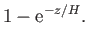Evaluate this fraction for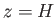,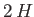,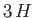, respectively.

2. If the absolute temperature in the atmosphere diminishes upwards according to the law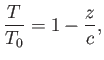where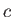is a constant, show that the pressure varies as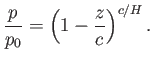3. If the absolute temperature in the atmosphere diminishes upward according to the law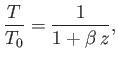where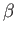is a constant, show that the pressure varies as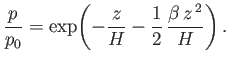4. Show that if the absolute temperature,, in the atmosphere is any given function of the altitude,, then the vertical distribution of pressure in the atmosphere is given by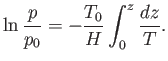5. Show that if the Earth were surrounded by an atmosphere of uniform temperature then the pressure a distancefrom the Earth's center would be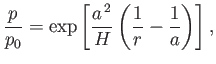where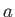is the Earth's radius.

6. Show that if the whole of space were occupied by air at the uniform temperaturethen the densities at the surfaces of the various planets would be proportional to the corresponding values of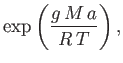whereis the radius of the planet, and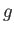its surface gravitational acceleration.

7. Prove that in an atmosphere arranged in horizontal strata the work (per unit mass) required to interchange two thin strata of equal mass without disturbance of the remaining strata is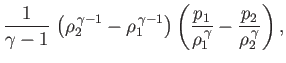where the suffixes refer to the initial states of the two strata. Hence, show that for stability the ratio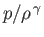must increase upwards.
8. A spherically symmetric star is such that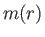is the mass contained within radius. Show that the star's total gravitational potential energy can be written in the following three alternative forms: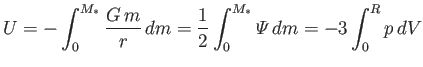Here,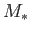is the total mass,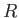the radius,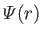the gravitational potential per unit mass (defined such that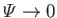as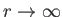),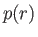the pressure, and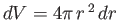.

9. Suppose that the pressure and density inside a spherically symmetric star are related according to the polytropic gas law,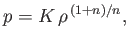where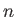is termed the polytropic index. Let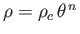, where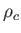is the central mass density. Demonstrate that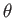satisfies the Lane-Emden equation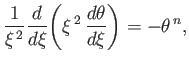where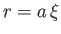, and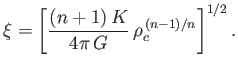Show that the physical solution to the Lane-Emden equation, which is such that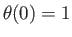and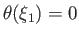, for some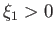, is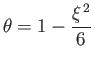for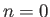,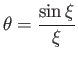for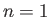, and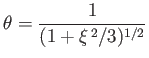for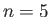. Determine the ratio of the central density to the mean density in all three cases. Finally, demonstrate that, in the general case, the total gravitational potential energy can be written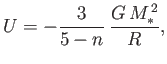whereis the total mass, and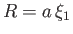the radius.
10. A spherically symmetric star of radiushas a mass density of the form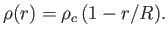Show that the central mass density is four times the mean density. Demonstrate that the central pressure is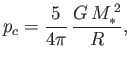whereis the mass of the star. Finally, show that the total gravitational potential energy of the star can be written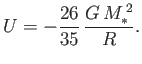Next: One-Dimensional Compressible Inviscid Flow Up: Equilibrium of Compressible Fluids Previous: Eddington Solar Model
Richard Fitzpatrick 2016-03-31